Shinco Environmental Technology Co., Ltd..

400-1892-315

# “煤改电”推动空气源热泵行业发展全屋采暖主机首选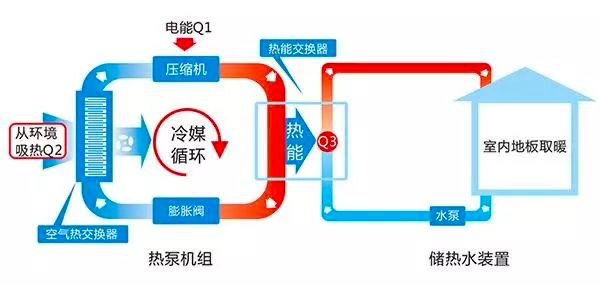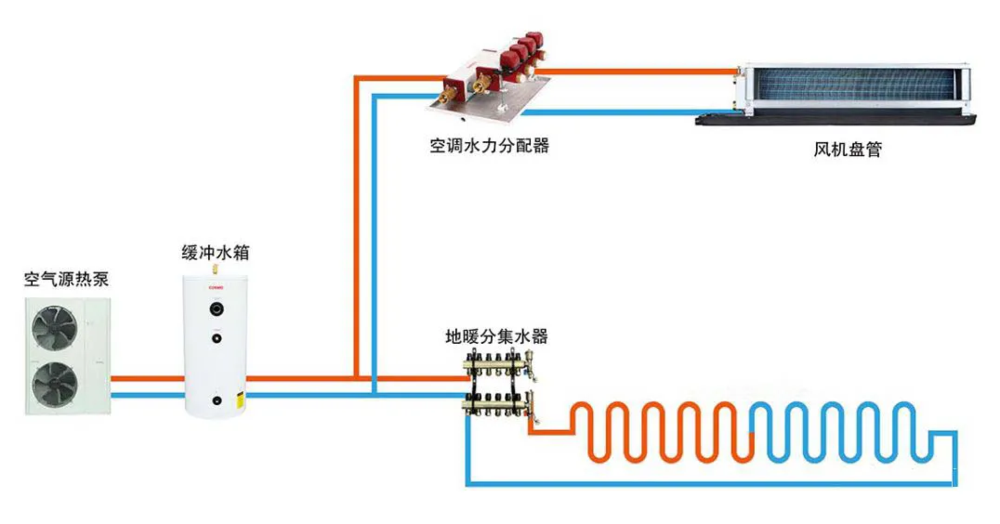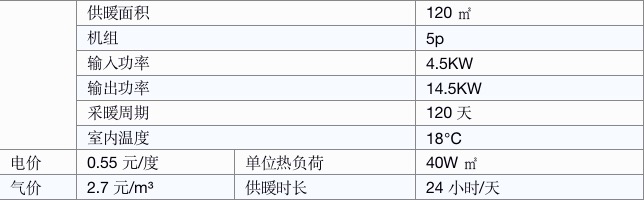Q=q×S×T×t×/1000=40×120×120×24/1000=13824kw。

Q：总热负荷值kW；q：单位热负荷值W/（㎡•h）；S：房间面积㎡；T：天数，天；t：供暖时长，h/天

Q=Q×1/2.8=13824/2.8=4937kw

L=Q/（36/3.6）=13824/10=1382.4m³

=Q1×1×0.55=4937×0.55=2715.4元

=L×2.7=1382.4×2.7=3722.6元。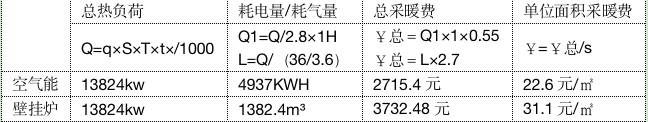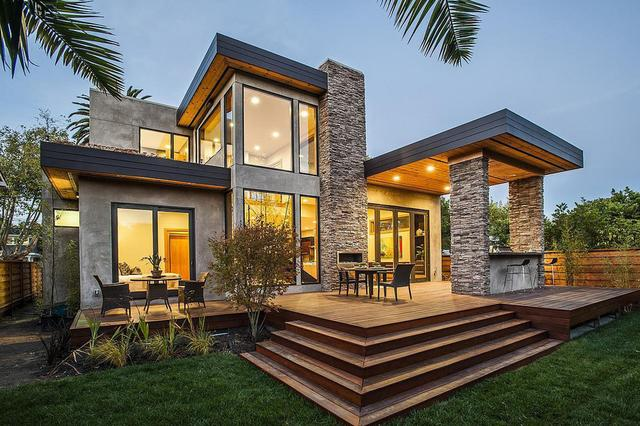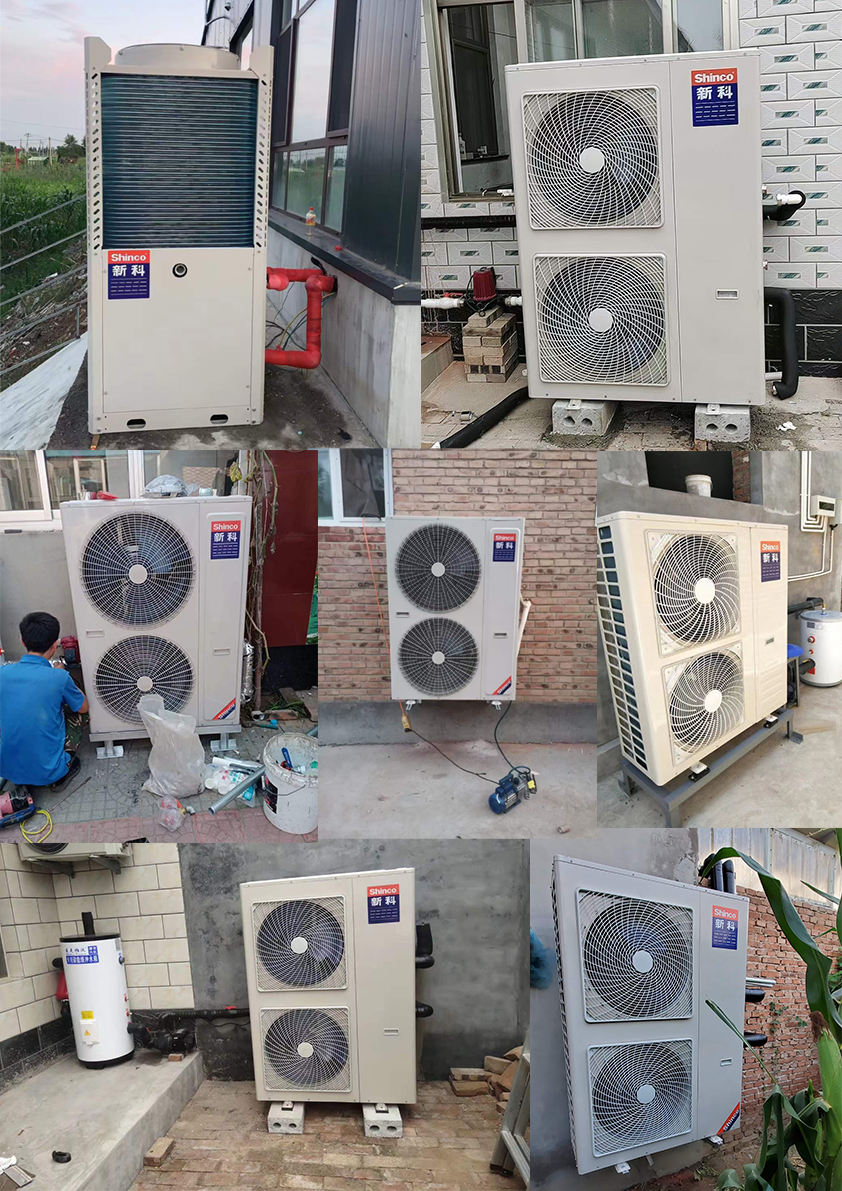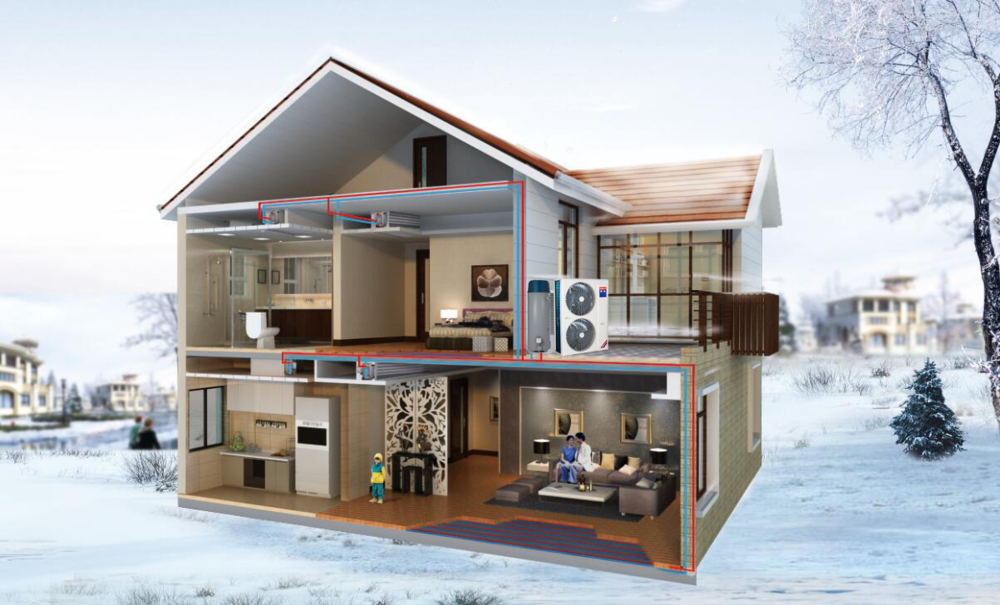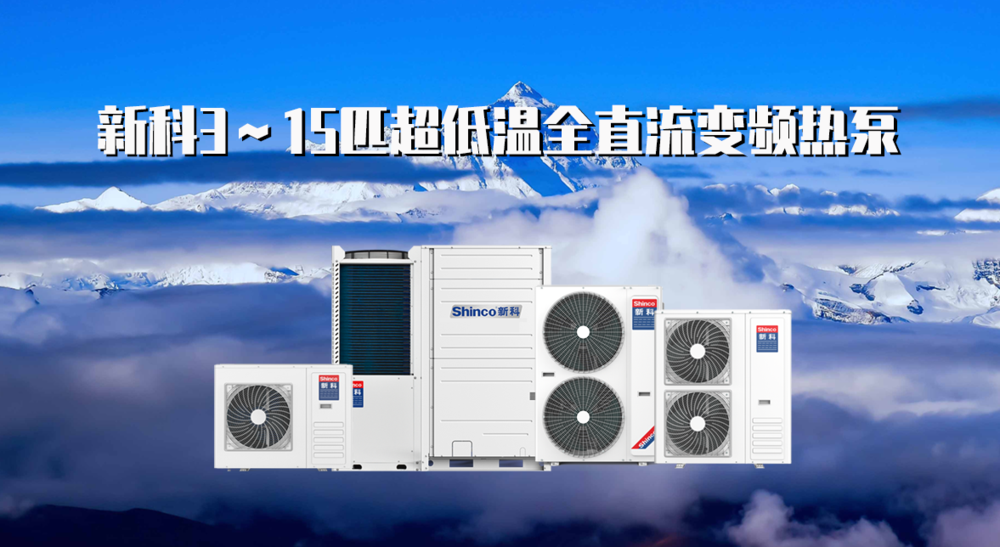400-1892-315